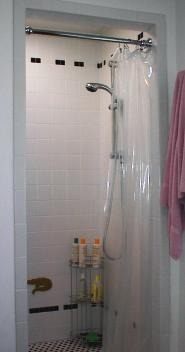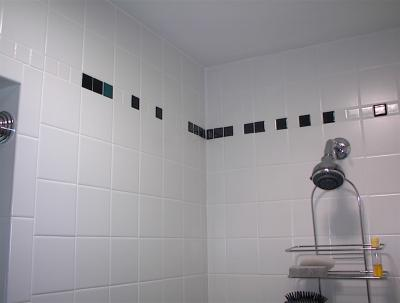# The pi-and-e Shower Stall

We installed a new bathroom in our house in summer 2000. We wanted a shower stall with tile walls and floor, with white tiles on the walls punctuated somehow for visual interest. Ordinarily, one might have as punctuation a row of tiles in a different color, or a pattern of some kind in multiple colors. We wanted the punctuation to be a bit unusual and to express our shared interest in mathematics, and came up with the idea of nonrepeating friezes based on the binary representations of the numbers pi and e.

Here's how it works. In decimal (base ten), the number 'pi', which is the ratio of a circle's circumference to its diameter, is written

3.1415926535...

In this representation, each digit to the right of the decimal point is divided by a power of ten that increases as you go to the right. So pi is 3 plus 1/10 plus 4/100 plus 1/1000 plus etc.

In binary (base 2), pi is represented as

11.001001000011111101101010100010...

In this representation, each bit (binary digit) to the right of the binary point is divided by a power of two that increases as you go to the right: we divide by 2, then 4, then 8, then 16, and so on. This means that pi is 2 plus 1 (in base 2, 11 is the number three) plus 0/2 plus 0/4 plus 1/8 plus 0/16 plus 0/32 plus 1/64 plus etc.

The number 'e' is much more important in mathematics than pi is, but isn't as familiar to most people. Let me just say that it's the number of dollars you would have after one year in an account that began with \$1 and that received interest at an annual rate of 100%, compounded continuously. Its value in decimal is

2.7182818284...

or, in binary,

10.101101111110000101010001011000...

Each frieze consists of a row of black and white tiles that starts to the right of the door and continues on around to each wall. The pi frieze is 8 inches below the ceiling, and the e frieze is 8 inches above the floor. White tiles correspond to 0 bits in the number's binary representation and black tiles correspond to 1 bits. The binary point shows up as a single teal-colored tile, and a few white (zero) tiles are thrown in at the beginning. So the pi frieze looks like

and the e frieze looks like

(except that the tiles are square).doorway into shower stallpiSomehow this page got noticed by reddit. And it inspired another shower.